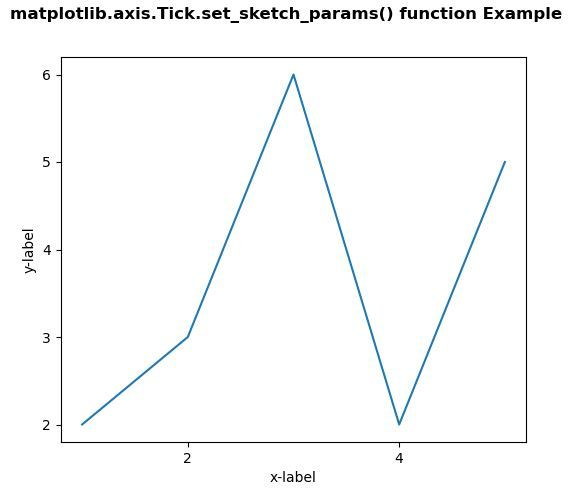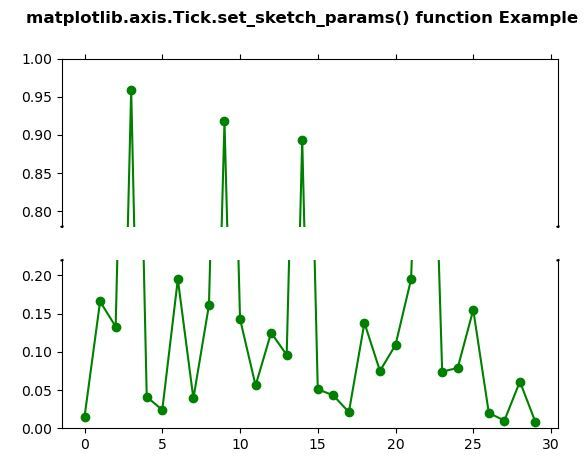Related Articles

# Matplotlib.axis.Tick.set_sketch_params() function in Python

• Last Updated : 10 Jun, 2020

Matplotlib is a library in Python and it is numerical – mathematical extension for NumPy library. It is an amazing visualization library in Python for 2D plots of arrays and used for working with the broader SciPy stack.

## Matplotlib.axis.Tick.set_sketch_params() Function

The Tick.set_sketch_params() function in axis module of matplotlib library is used to sets the sketch parameters.

Syntax: Tick.set_sketch_params(self, scale=None, length=None, randomness=None)
Parameters: This method accepts the following parameters.

• scale: This parameter is the amplitude of the wiggle perpendicular to the source line, in pixels.
• length: This parameter is the length of the wiggle along the line, in pixels.
• randomness: This parameter is the scale factor by which the length is shrunken or expanded.

Return value: This method does not return any value.

Below examples illustrate the matplotlib.axis.Tick.set_sketch_params() function in matplotlib.axis:
Example 1:

## Python3

 `# Implementation of matplotlib function``from` `matplotlib.axis ``import` `Tick``import` `matplotlib.pyplot as plt  ``import` `matplotlib.colors as mcolors  ``import` `matplotlib.gridspec as gridspec  ``import` `numpy as np  ``        ` `        ` `plt.rcParams[``'savefig.facecolor'``] ``=` `"0.8"``plt.rcParams[``'figure.figsize'``] ``=` `6``, ``5``        ` `fig, ax ``=` `plt.subplots()  ``        ` `ax.plot([``1``, ``2``, ``3``, ``4``, ``5``] , [``2``, ``3``,``6``,``2``,``5``])  ``        ` `ax.locator_params(``"x"``, nbins ``=` `3``)  ``ax.locator_params(``"y"``, nbins ``=` `5``)  ``        ` `ax.set_xlabel(``'x-label'``)  ``ax.set_ylabel(``'y-label'``)  ``      ` `Tick.set_sketch_params(ax, ``50``, ``50``, ``10``)`` ` `fig.suptitle('matplotlib.axis.Tick.set_sketch_params() \``function Example', fontweight ``=``"bold"``)  ``    ` `plt.show() `

Output:Example 2:

## Python3

 `# Implementation of matplotlib function``from` `matplotlib.axis ``import` `Tick``import` `matplotlib.pyplot as plt  ``import` `numpy as np  ``       ` `values ``=` `np.array([  ``    ``0.015``, ``0.166``, ``0.133``,   ``    ``0.159``, ``0.041``, ``0.024``,  ``    ``0.195``, ``0.039``, ``0.161``,  ``    ``0.918``, ``0.143``, ``0.056``,  ``    ``0.125``, ``0.096``, ``0.094``,  ``    ``0.051``, ``0.043``, ``0.021``,  ``    ``0.138``, ``0.075``, ``0.109``,  ``    ``0.195``, ``0.750``, ``0.074``,   ``    ``0.079``, ``0.155``, ``0.020``,  ``    ``0.010``, ``0.061``, ``0.008``])  ``       ` `values[[``3``, ``14``]] ``+``=` `.``8``       ` `fig, (ax, ax2) ``=` `plt.subplots(``2``, ``1``, sharex ``=` `True``)  ``       ` `ax.plot(values, ``"o-"``, color ``=``"green"``)  ``ax2.plot(values, ``"o-"``, color ``=``"green"``)  ``       ` `ax.set_ylim(.``78``, ``1.``)   ``ax2.set_ylim(``0``, .``22``)  ``       ` `ax.spines[``'bottom'``].set_visible(``False``)  ``ax2.spines[``'top'``].set_visible(``False``)  ``ax.xaxis.tick_top()  ``ax.tick_params(labeltop ``=` `False``)  ``ax2.xaxis.tick_bottom()  ``       ` `d ``=` `.``001``kwargs ``=` `dict``(transform ``=` `ax.transAxes,   ``              ``color ``=``'k'``, clip_on ``=` `False``)  ``      ` `ax.plot((``-``d, ``+``d), (``-``d, ``+``d), ``*``*``kwargs)         ``ax.plot((``1` `-` `d, ``1` `+` `d), (``-``d, ``+``d), ``*``*``kwargs)   ``       ` `kwargs.update(transform ``=` `ax2.transAxes)    ``      ` `ax2.plot((``-``d, ``+``d), (``1` `-` `d, ``1` `+` `d), ``*``*``kwargs)  ``ax2.plot((``1` `-` `d, ``1` `+` `d), (``1` `-` `d, ``1` `+` `d), ``*``*``kwargs)   ``    ` `Tick.set_sketch_params(ax, ``1.0``, ``10.0``, ``25.0``)  ``Tick.set_sketch_params(ax2, ``2.0``, ``100.0``, ``50.0``) `` ` `fig.suptitle('matplotlib.axis.Tick.set_sketch_params() \``function Example', fontweight ``=``"bold"``)  ``    ` `plt.show() `

Output:Attention geek! Strengthen your foundations with the Python Programming Foundation Course and learn the basics.

To begin with, your interview preparations Enhance your Data Structures concepts with the Python DS Course. And to begin with your Machine Learning Journey, join the Machine Learning – Basic Level Course

My Personal Notes arrow_drop_up